• OPENCV 图像显示视频课程介绍。主要是学习的代码类。结合visual c++6.0来进行详细的介绍
• opencv上用C/C++代码编写的用于读入和输出图像的代码，适合opencv初学者学习
• opencv 读取图像像素值到数组，用指针方式实现 opencv 读取图像像素值到数组，用指针方式实现
• opencv获取图像像素值,学习opencv如何读图片并显示出来，获取像素值
• ## opencv读取图像失败

千次阅读 2014-03-06 20:25:32
使用vs2012+opencv读取图像，确定图像路径和图像格式没问题，但是总是读不进来。 原因：在设置附加依赖项时，将debug时使用的×.lib的d忘加了。 应使用opencv_highgui248d.lib,用成了opencv_highgui248.lib
使用vs2012+opencv读取图像，确定图像路径和图像格式没问题，但是总是读不进来。
原因：在设置附加依赖项时，将debug时使用的×.lib的d忘加了。
应使用opencv_highgui248d.lib,用成了opencv_highgui248.lib
展开全文debug
• 引言opencv是计算机视觉中使用最广泛同时也是功能最全的一个开源库，为图像处理以及计算机视觉工作者提供了极大的方便，本文就opencv读取图像数据文件做一个总结，高年级同学以及大牛请无视。opencv中图像的结构图像...
引言
opencv是计算机视觉中使用最广泛同时也是功能最全的一个开源库，为图像处理以及计算机视觉工作者提供了极大的方便，本文就opencv读取图像数据文件做一个总结，高年级同学以及大牛请无视。
opencv中图像的结构
图像的结构可以看作是一个2维矩阵，opencv在对图像的结构定义中也采用了这一方式。在总结之前，有必要对opencv中图像结构定义的一些成员变量意义进行说明，更详细的请参考opencv官方文档。  nChannels：图像通道数，可取1、2、3、4；  widthStep：可理解成相邻行，同列数据之间的字节数，为了高速的处理效率，图像在存储时会进行填充，保证每行是一个固定字节数，且通常维4的倍数；  width：图像宽；  height：图像高；
数据读取
1.点读取
// 单通道
uchar value = grayim.at<uchar>(i,j);
for( int i = 0; i < grayim.rows; ++i)
for( int j = 0; j < grayim.cols; ++j )
grayim.at<uchar>(i,j) = (i+j)%255;

//3通道 Vec3b可看作一个结构体，包含三个元素，分别对应彩色图像的B\G\R，且只能对8U的数据类型使用，如果是float数据的值，可以用Vec3f

for( int i = 0; i < colorim.rows; ++i)
for( int j = 0; j < colorim.cols; ++j )
{
Vec3b pixel;
pixel = i%255; //Blue
pixel = j%255; //Green
pixel = 0; //Red
colorim.at<Vec3b>(i,j) = pixel;
}
2.图像迭代器
// 单通道
cv::MatIterator_<uchar> grayit, grayend;
for( grayit = grayim.begin<uchar>(), grayend =
grayim.end<uchar>(); grayit != grayend; ++grayit)
*grayit = rand()%255;

//3通道
cv::MatIterator_<Vec3b> colorit, colorend;
for( colorit = colorim.begin<Vec3b>(), colorend = colorim.end<Vec3b>(); colorit!=colorend; colorit++)
{
(*colorit)=rand()%255; //B
(*colorit)=rand()%255; //G
(*colorit)=rand()%255; //R
}
3.指针读取
for( int i = 0; i < grayim.rows; ++i)
{
//获取第 i 行首像素指针
uchar * p = grayim.ptr<uchar>(i);
//对第 i 行的每个像素(byte)操作
for( int j = 0; j < grayim.cols; ++j )
p[j] = (i+j)%255;
}
4.按结构读取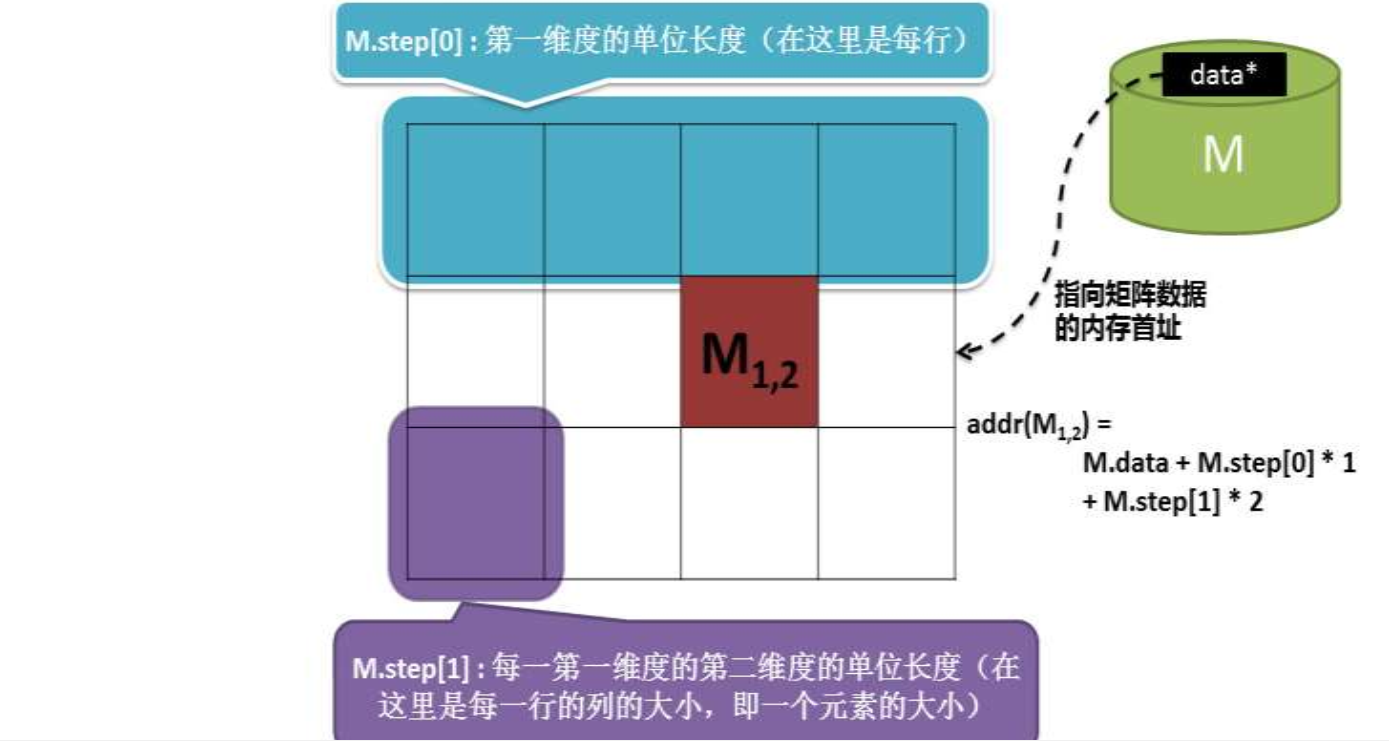addr(Mi0,i1,…im-1) = M.data + M.step * i0 + M.step * i1 + … +
M.step[m-1] * im-1 (其中 m = M.dims M的维度)
扩展到3维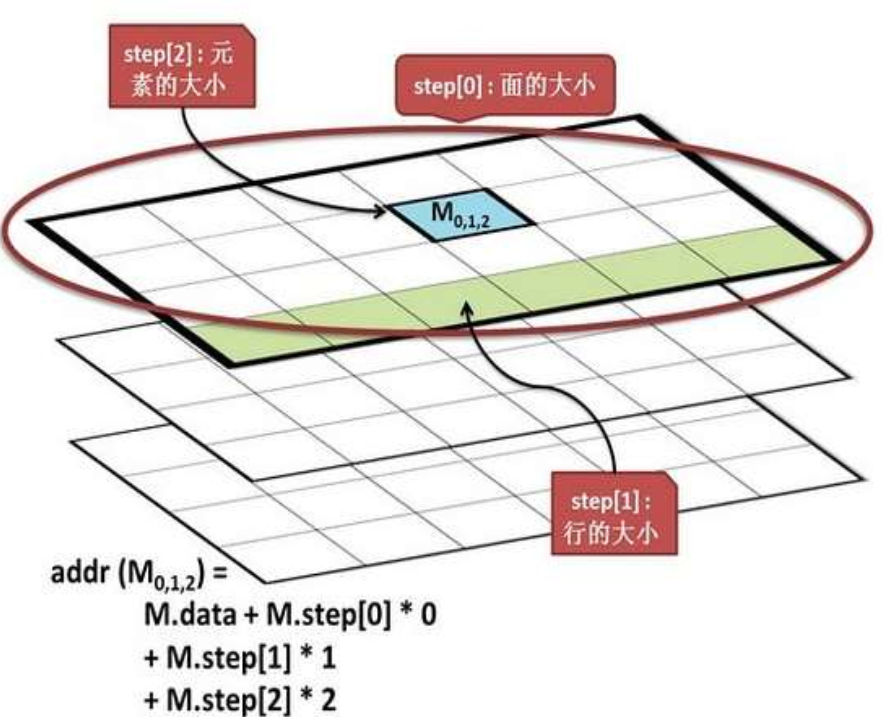单通道：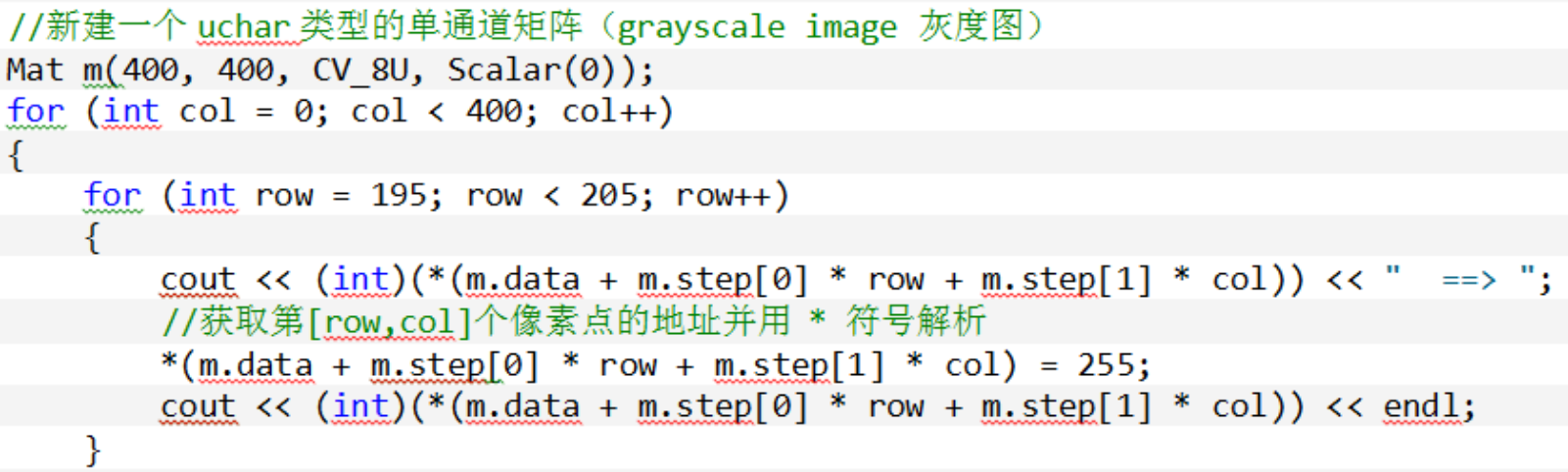3通道：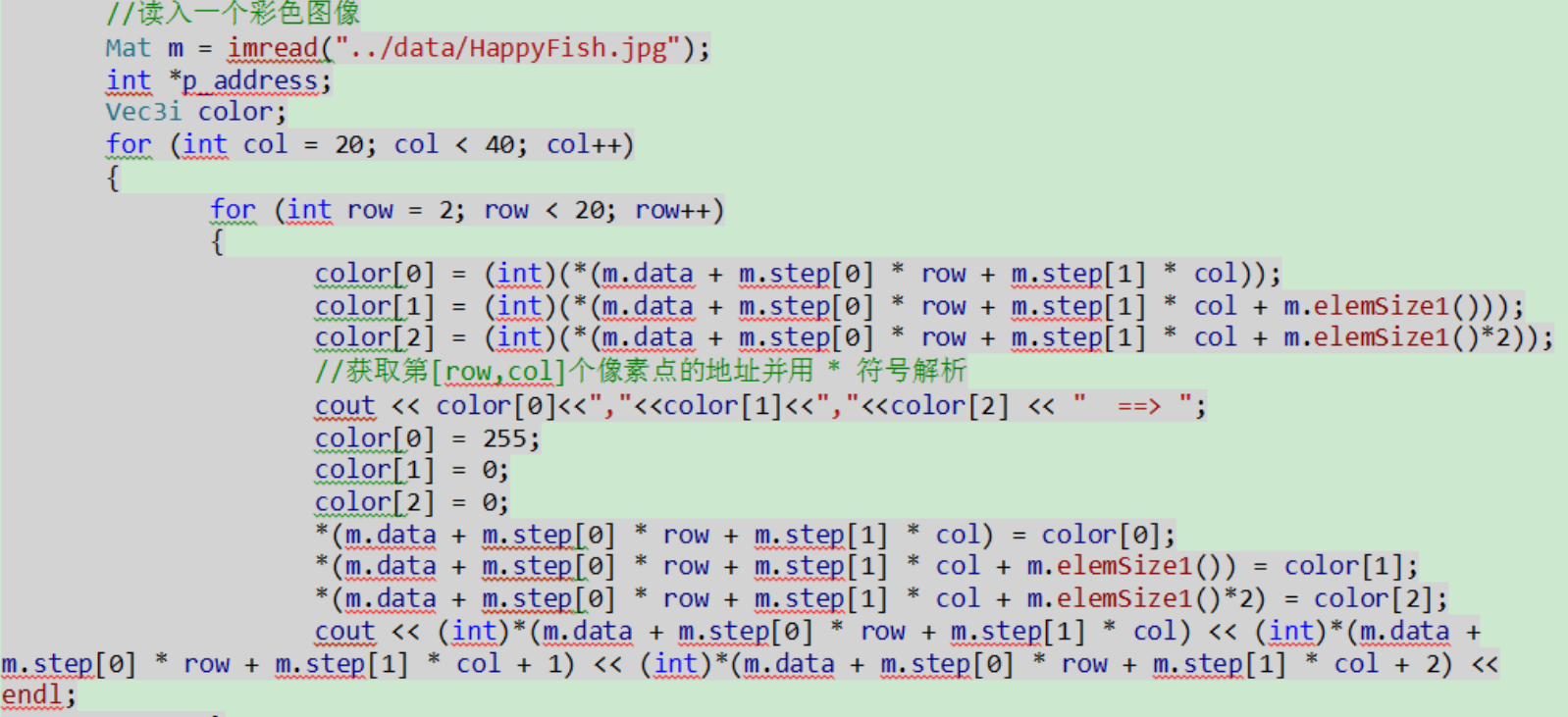5.Mat_读取
考虑下面一段代码：
// 由于数据类型转换，最后的像素值会发生改变
Mat M(600, 800, CV_8UC1);
for( int i = 0; i < M.rows; ++i)
{
uchar * p = M.ptr<uchar>(i);
for( int j = 0; j < M.cols; ++j )
{
double d1 = (double) ((i+j)%255);
M.at<uchar>(i,j) = d1;
double d2 = M.at<double>(i,j);
}
}
如果采用Mat_模板类则可以避免这种情况：
Mat_<uchar> M1 = (Mat_<uchar>&)M; // 内存地址共享
for( int i = 0; i < M1.rows; ++i)
{
uchar * p = M1.ptr(i);
for( int j = 0; j < M1.cols; ++j )
{
double d1 = (double) ((i+j)%255);
M1(i,j) = d1;
double d2 = M1(i,j);
}
}
展开全文计算机视觉
• 利用opencv自带函数，读取一个图像的通道数，之前一直出错，原来是自己设置错了
• python用opencv读取图像如何做格式转换 在python中用cv2读取图像文件或摄像头，都能获取一帧图像，如 import cv2 frame1 = cv2.imread(“a.jpg”) cap = cv2.VideoCapture(0) rval, frame2 = cap.read() ...
python用opencv读取图像如何做格式转换
在python中用cv2读取图像文件或摄像头，都能获取一帧图像，如

import cv2  frame1 = cv2.imread(“a.jpg”)  cap = cv2.VideoCapture(0)  rval, frame2 = cap.read()

上面获得的frame1和frame2都是numpy array格式的。但是有时候我们想要把它转成自己的格式，比如有时候可能需要调用C++函数，比如在darknet中，这时就要进行格式转换。这个方法有如下几种：
存成文件，再用相应的读文件方法读取  这种方法这里不谈，毕竟频繁地读写文件的方法在很多场合下是不可取的，其实速度还可以，但仍然不是一种完美的方式。直接在python中做格式转换  既然知道frame是numpy array格式，当然可以直接做转换，但是在python中进行这种操作非常耗时。笔者测试了一下，转换一张416x416x3的图像大约需要3秒钟。这个速度甚至不如用文件转存的方式。本文重点要说的，是通过C函数进行转换  首先，从numpy array获得C数据指针：

h,w,c=frame1.shape  if not frame1.flags[‘C_CONTIGUOUS’]:  frame1= np.ascontiguousarray(frame1, dtype=frame1.dtype) # 转换成连续存放  data_ptr = cast(frame1.ctypes.data, c_char_p)
这个data_ptr 就是所要传递给C函数的数据指针，此外还得到了图像高度h，宽度w和通道数c。 下面要做的，是在python中声明一个自己定义的C函数mat2local_image，比如在darknet中：

lib = CDLL(“libdarknet.so”, RTLD_GLOBAL)  mat2local_image = lib.mat2local_image  mat2local_image.argtypes = [c_int,c_int,c_int,c_char_p]  mat2local_image.restype = IMAGE
其中IMAGE是自己定义的数据格式，比如在darknet中是这样定义的：

class IMAGE(Structure):  fields = [(“w”, c_int),  (“h”, c_int),  (“c”, c_int),  (“data”, POINTER(c_float))]
此外，你还要在C文件中定义一个C函数mat2local_image，例如在darknet中：

image mat2local_image(int w, int h, int c, unsigned char *data)  {  int i, j, k;  image im = make_image(w, h, c);  for(i = 0; i < h; ++i){  for(k= 0; k < c; ++k){  for(j = 0; j < w; ++j){//data is BGR, so c-k-1 to reverse to RGB  im.data[k*w*h + i*w + j] = data[i*w*c + j*c + c-k-1]/255.;  }  }  }  //It has been reversed to RGB  //rgbgr_image(im);  return im;  }
注意，因为cv2读图像的格式是BGR，所以在转换时，需要将R,B交换。在这个函数中，我们直接通过c-k-1进行这个交换，而不需要调用rgbgr_image函数。然后把C文件编译成.so。 现在，你可以在python中调用这个函数了：

def image_mat2local(image_mat):  h,w,c=frame1.shape  if not frame1.flags[‘C_CONTIGUOUS’]:  frame1= np.ascontiguousarray(frame1, dtype=frame1.dtype) # 转换成连续存放  data_ptr = cast(frame1.ctypes.data, c_char_p)  im = mat2local_image(w,h,c,data_ptr)  return im

请关注爱在上工：展开全文python 计算机视觉 darknet
• opencv图片格式是按（宽和高）的形式 tensorflow是以数据行，列的逻辑 opencv图片的宽高逻辑 所以在读取文件，训练，resize的时候一定要注意 例如： 这里的（80，120）是高和宽 而在opencv里需要这么反着写：...
这里有个坑！
tensorflow的图片格式是按（高，宽）的形式
而opencv的图片格式是按（宽和高）的形式
tensorflow是以数据行，列的逻辑
opencv是图片的宽高逻辑
所以在读取文件，训练，resize的时候一定要注意 例如：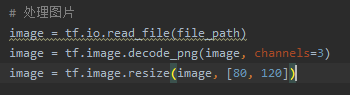这里的（80，120）是高和宽 而在opencv里需要这么反着写：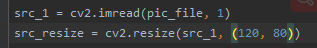在构建神经网络时就一定得注意，是按照rows, cols的逻辑来，也就是高和宽，下面就写错了：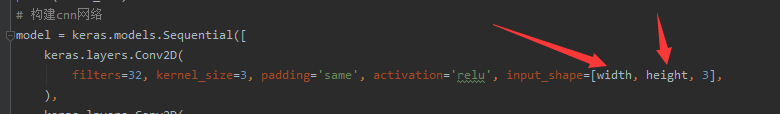展开全文• imread:从字面意思我们就可以看懂，用来读取图片的； namedWindow:显然，我们也可以看到这是用来命名窗口名称的； imshow:这个自然就是来显示窗口的，窗口内的当然就是要显示的图片了。 代码来自OpenCV Tutorials 这...python 深度学习
• 下面小编就为大家带来一篇python使用opencv读取图片的实例。小编觉得挺不错的，现在就分享给大家，也给大家做个参考。一起跟随小编过来看看吧
• 使用cv2.imread()函数可以读取图片的高，宽，通道数。奇异返回值的第一个值就是图像的高度。 具体代码如下： import cv2 as cv import numpy as np im=cv.imread('fruit.png') height=im.shape cv.imshow('...python
• python调用opencv读取图片（二）1、在Viual Studio 中运行python读取图片2、编写一个opencv读取图片的程序 1、在Viual Studio 中运行python读取图片 在Visual Studio 中配置python开发环境 配置如图所示： 上述...
• QT OPENCV读取图片一直读取不成功 我用c语言版本的qt和opencv读取图片，视频可以正常读取，但是图片就是不行，图片路径也是对的，后面发现，我用的debug模式，但是链接库的时候，把debug和release中的库都链接进来了...
• OpenCv 获取图像联通域具体分析 VC的具体实现，有很大的参考价值
• 用鼠标取图片中的像素值#include<opencv2\opencv.hpp> #include using namespace cv; using namespace std; bool flag = false; int xvalue = 0; int yvalue = 0; Mat image,image1,image2; void mousecallback(int ...
• OpenCV读取图像显示在MFC窗口上  1. 在MFC上创建一个图片控件，自己修改ID，比如IDC_PICTURE,并且添加对应的变量 CStatic m_PictureControl  2. 在打开图片的按钮触发事件中添加如下代码： namedWindow...
• 使用opencv读取图片时出现错误：cv2.error: OpenCV(4.4.0)解决办法 - 利用opencv对图像进行增强时出现如下错误： cv2.error: OpenCV(4.4.0) C:\Users\appveyor\AppData\Local\Temp\1\pip-req-build-k8sx3e60\opencv\...python 计算机视觉
• pp.jpg须存在工程目录下。opencv得预先费心配置好
• 主要介绍了使用OpenCV获取图像某点的颜色值,并设置某点的颜色，具有很好的参考价值，希望对大家有所帮助。一起跟随小编过来看看吧
• 以下用OpenCV实现获取图像中某点的颜色值，并设置某点区域的颜色，OpenCV获取图像某点的颜色值，并设置某点的颜色
• #include <iostream>...opencv2/opencv.hpp> using namespace std; using namespace cv; int main() { const char *f0 = "E:/00opencv/img_data/7.jpg"; // 路径 Mat IMG = imread(f0, 1)...c++
• 其实并不是失真，而是在导入图片时使用了opencv， 正常展示顺序是RGB，而opencv读取顺序是BGR， 所以蓝色和红色的通道反了 因此在读取图像前面加两行转换一下即可。 完整代码如下 import cv2 from ...python
• Python 技术篇-使用opencv读取图片实例演示，python安装opencv库。 安装非常简单，只需要pip install opencv-python就好了。 下面展示用 opencv 读取一张图片，并展示。 #!/user/bin/env python # -*- coding:utf-8 ...opencv环境安装...